# The Basic Tools of Finance 9 Finance Finance

• Slides: 56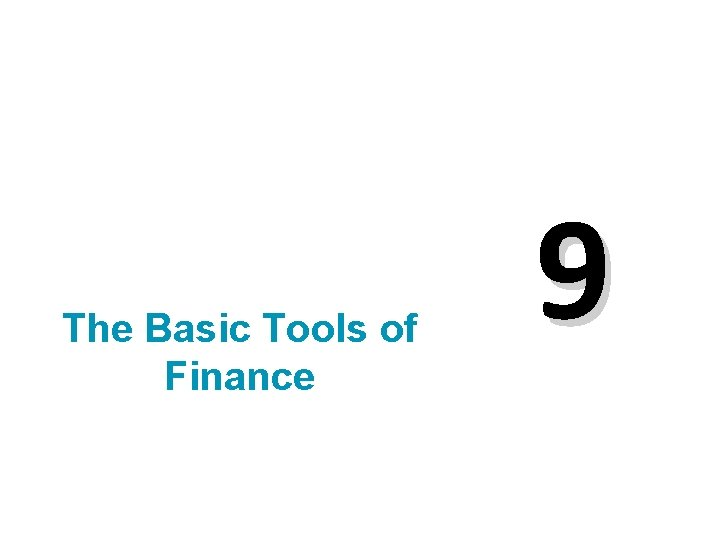The Basic Tools of Finance 9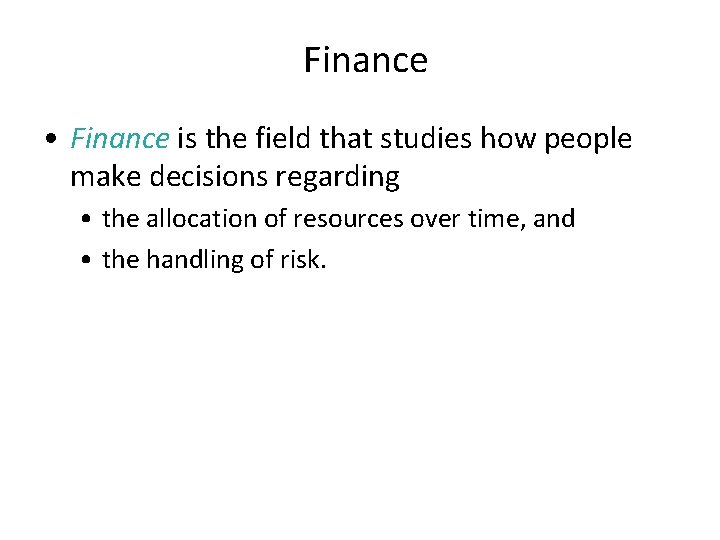Finance • Finance is the field that studies how people make decisions regarding • the allocation of resources over time, and • the handling of risk.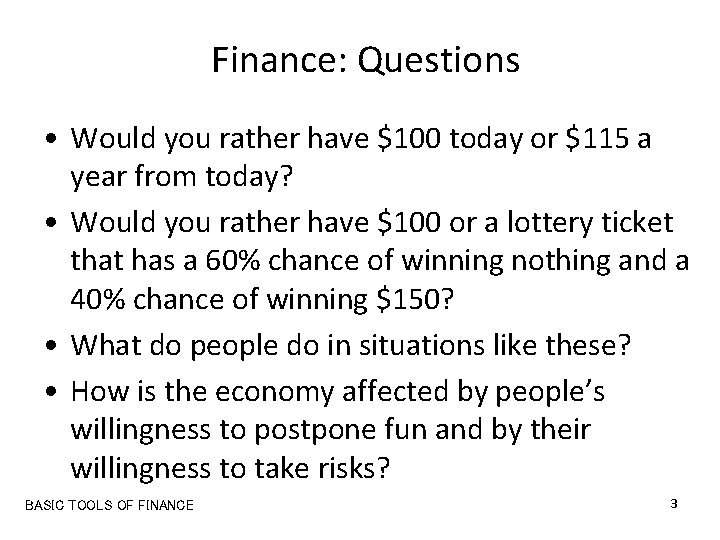Finance: Questions • Would you rather have \$100 today or \$115 a year from today? • Would you rather have \$100 or a lottery ticket that has a 60% chance of winning nothing and a 40% chance of winning \$150? • What do people do in situations like these? • How is the economy affected by people’s willingness to postpone fun and by their willingness to take risks? BASIC TOOLS OF FINANCE 3 Copyright © 2004 South-Western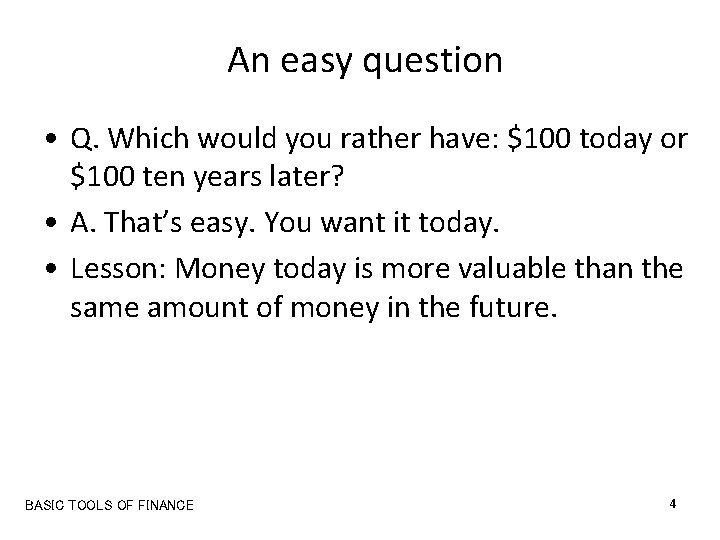An easy question • Q. Which would you rather have: \$100 today or \$100 ten years later? • A. That’s easy. You want it today. • Lesson: Money today is more valuable than the same amount of money in the future. BASIC TOOLS OF FINANCE 4 Copyright © 2004 South-Western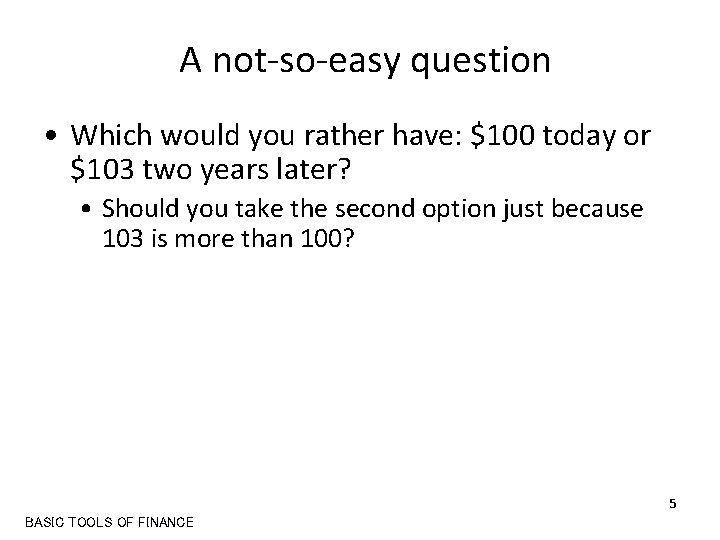A not-so-easy question • Which would you rather have: \$100 today or \$103 two years later? • Should you take the second option just because 103 is more than 100? 5 BASIC TOOLS OF FINANCE Copyright © 2004 South-Western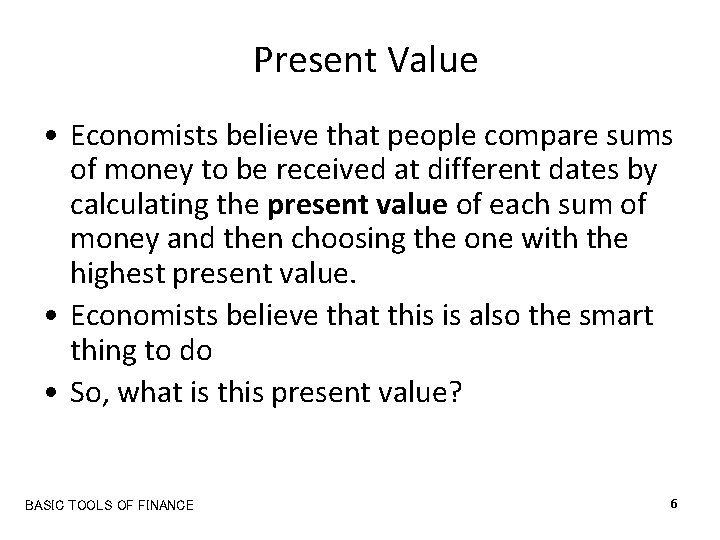Present Value • Economists believe that people compare sums of money to be received at different dates by calculating the present value of each sum of money and then choosing the one with the highest present value. • Economists believe that this is also the smart thing to do • So, what is this present value? BASIC TOOLS OF FINANCE 6 Copyright © 2004 South-Western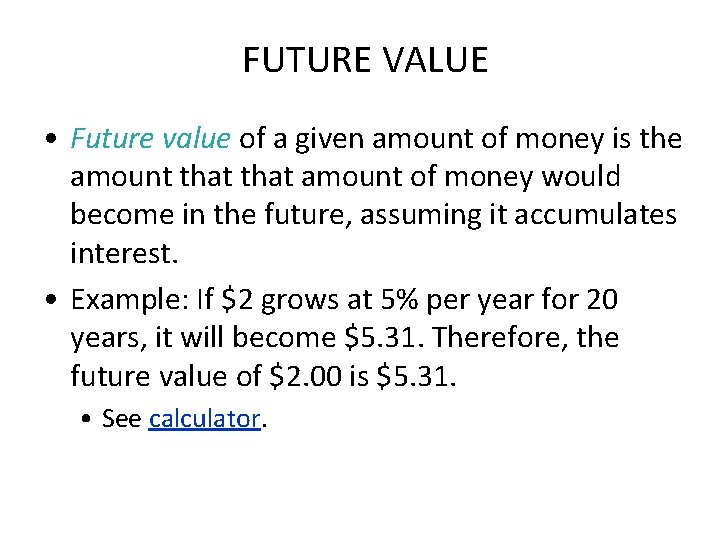FUTURE VALUE • Future value of a given amount of money is the amount that amount of money would become in the future, assuming it accumulates interest. • Example: If \$2 grows at 5% per year for 20 years, it will become \$5. 31. Therefore, the future value of \$2. 00 is \$5. 31. • See calculator.PRESENT VALUE: MEASURING THE TIME VALUE OF MONEY • Present value of a given future amount of money is the amount of money today that would grow, at prevailing interest rates, to the given future amount of money. • Example: If \$2 grows at 5% per year for 20 years, it will become \$5. 31. Therefore, the 20 year present value of \$5. 31 is \$2. • See calculator.Choices • Suppose you know that \$2. 00 will become \$5. 31 after 20 years. • That is, \$2. 00 is the present value of \$5. 31 received 20 years later • If you had to choose between \$2. 00 today and \$5. 31 twenty years later, which would you choose? BASIC TOOLS OF FINANCE 9 Copyright © 2004 South-Western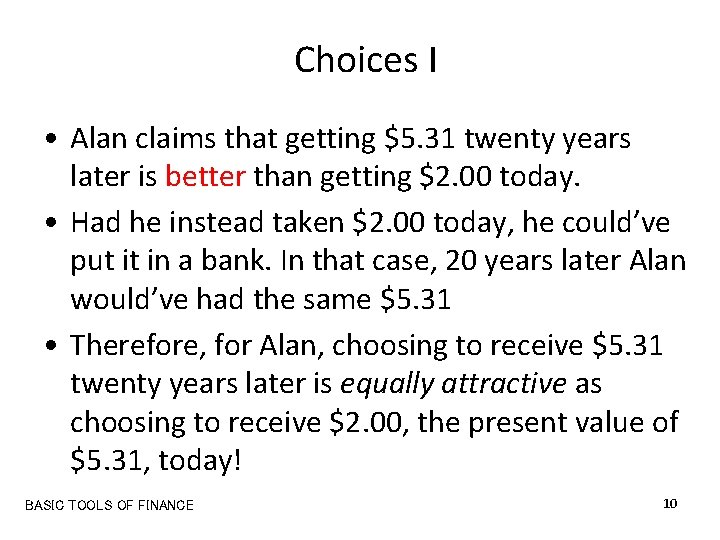Choices I • Alan claims that getting \$5. 31 twenty years later is better than getting \$2. 00 today. • Had he instead taken \$2. 00 today, he could’ve put it in a bank. In that case, 20 years later Alan would’ve had the same \$5. 31 • Therefore, for Alan, choosing to receive \$5. 31 twenty years later is equally attractive as choosing to receive \$2. 00, the present value of \$5. 31, today! BASIC TOOLS OF FINANCE 10 Copyright © 2004 South-Western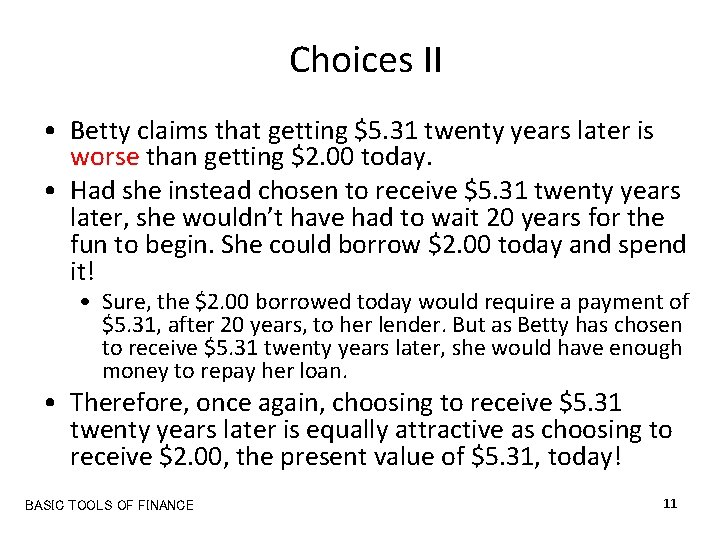Choices II • Betty claims that getting \$5. 31 twenty years later is worse than getting \$2. 00 today. • Had she instead chosen to receive \$5. 31 twenty years later, she wouldn’t have had to wait 20 years for the fun to begin. She could borrow \$2. 00 today and spend it! • Sure, the \$2. 00 borrowed today would require a payment of \$5. 31, after 20 years, to her lender. But as Betty has chosen to receive \$5. 31 twenty years later, she would have enough money to repay her loan. • Therefore, once again, choosing to receive \$5. 31 twenty years later is equally attractive as choosing to receive \$2. 00, the present value of \$5. 31, today! BASIC TOOLS OF FINANCE 11 Copyright © 2004 South-WesternChoice and present value • Therefore, whichever way you look at it, the prospect of receiving a given amount of money in the future is as attractive as receiving today the present value of that amount. BASIC TOOLS OF FINANCE 12 Copyright © 2004 South-Western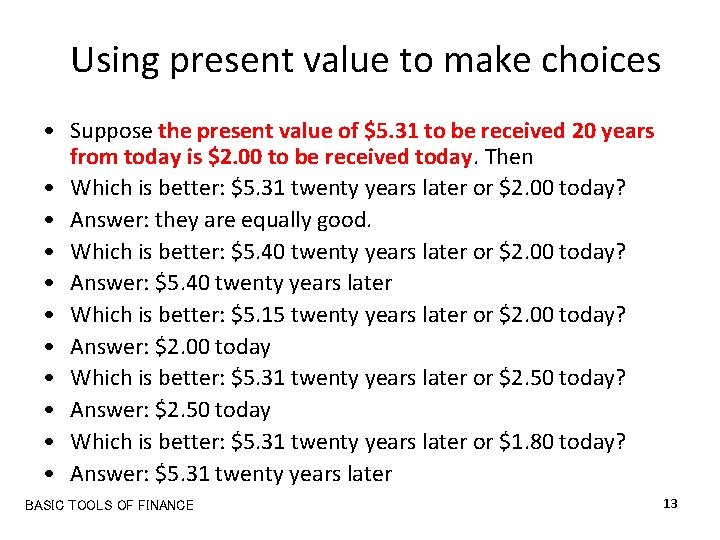Using present value to make choices • Suppose the present value of \$5. 31 to be received 20 years from today is \$2. 00 to be received today. Then • Which is better: \$5. 31 twenty years later or \$2. 00 today? • Answer: they are equally good. • Which is better: \$5. 40 twenty years later or \$2. 00 today? • Answer: \$5. 40 twenty years later • Which is better: \$5. 15 twenty years later or \$2. 00 today? • Answer: \$2. 00 today • Which is better: \$5. 31 twenty years later or \$2. 50 today? • Answer: \$2. 50 today • Which is better: \$5. 31 twenty years later or \$1. 80 today? • Answer: \$5. 31 twenty years later BASIC TOOLS OF FINANCE 13 Copyright © 2004 South-WesternUsing present value to make choices • Suppose the present value of \$5. 31 to be received 20 years from today is \$2. 00 to be received today. Then • Which is better: \$200 twenty years later or \$80 today? • We know that \$5. 31 twenty years later is equally attractive as \$2. 00 today. Therefore, • \$5. 31 × 40 twenty years later must be equally attractive as \$2. 00 × 40 today. • Therefore, \$212. 40 twenty years later must be equally attractive as \$80 today. Therefore • Answer: \$80 today is better than \$200 twenty years later. • In this way, any amount receivable 20 years later can be compared to any amount receivable today BASIC TOOLS OF FINANCE 14 Copyright © 2004 South-WesternChoices and present value: examples • You have to choose between • \$428. 72 after 8 years, • \$659. 12 after 12 years, and • \$1379. 14 after 20 years. • Which would you choose? • Suppose the present values of your three choices are \$200, \$210, and \$205, respectively. That is, • \$200 will become \$428. 72 after 8 years, • \$210 will become \$659. 12 after 12 years, and • \$205 will become \$1379. 14 after 20 years. • This happens if the interest rate is 10%. See calculator. BASIC TOOLS OF FINANCE 16 Copyright © 2004 South-Western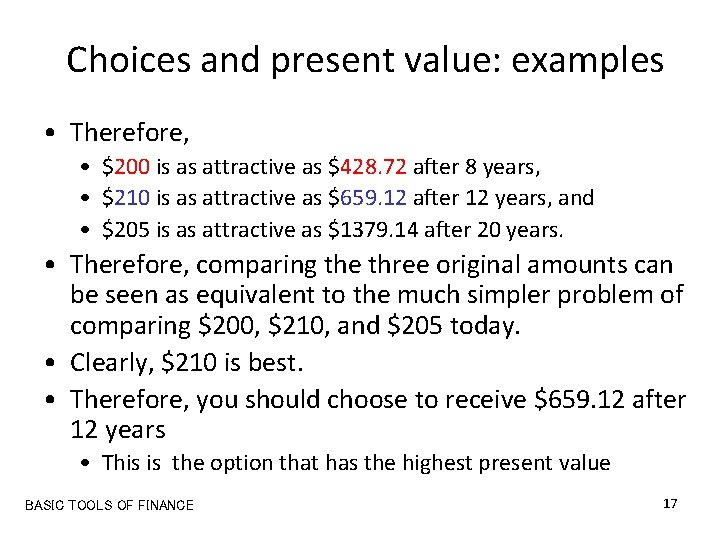Choices and present value: examples • Therefore, • \$200 is as attractive as \$428. 72 after 8 years, • \$210 is as attractive as \$659. 12 after 12 years, and • \$205 is as attractive as \$1379. 14 after 20 years. • Therefore, comparing the three original amounts can be seen as equivalent to the much simpler problem of comparing \$200, \$210, and \$205 today. • Clearly, \$210 is best. • Therefore, you should choose to receive \$659. 12 after 12 years • This is the option that has the highest present value BASIC TOOLS OF FINANCE 17 Copyright © 2004 South-Western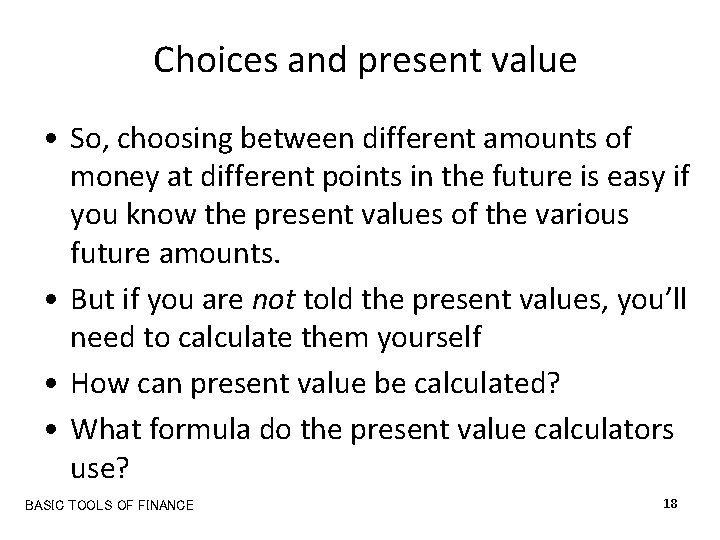Choices and present value • So, choosing between different amounts of money at different points in the future is easy if you know the present values of the various future amounts. • But if you are not told the present values, you’ll need to calculate them yourself • How can present value be calculated? • What formula do the present value calculators use? BASIC TOOLS OF FINANCE 18 Copyright © 2004 South-Western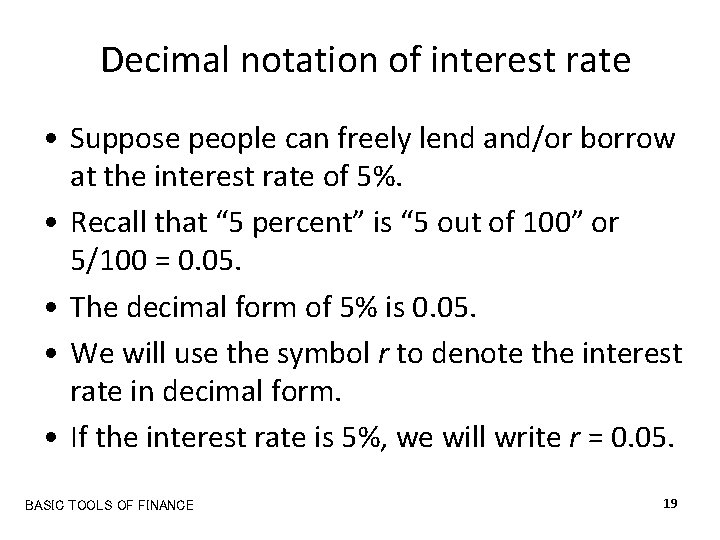Decimal notation of interest rate • Suppose people can freely lend and/or borrow at the interest rate of 5%. • Recall that “ 5 percent” is “ 5 out of 100” or 5/100 = 0. 05. • The decimal form of 5% is 0. 05. • We will use the symbol r to denote the interest rate in decimal form. • If the interest rate is 5%, we will write r = 0. 05. BASIC TOOLS OF FINANCE 19 Copyright © 2004 South-WesternCompounding: One year • If r = 0. 05, then \$200 deposited in a bank today will grow in one year to: • The original amount (or, principal) of \$200, plus • The interest earned, which is 0. 05 × 200 = \$10. • That is, if r = 0. 05, then \$200 will grow in one year to 200 + 0. 05 × 200 = (1 + 0. 05) × 200 = \$210 • Suppose this amount is immediately reinvested for yet another year. BASIC TOOLS OF FINANCE 20 Copyright © 2004 South-Western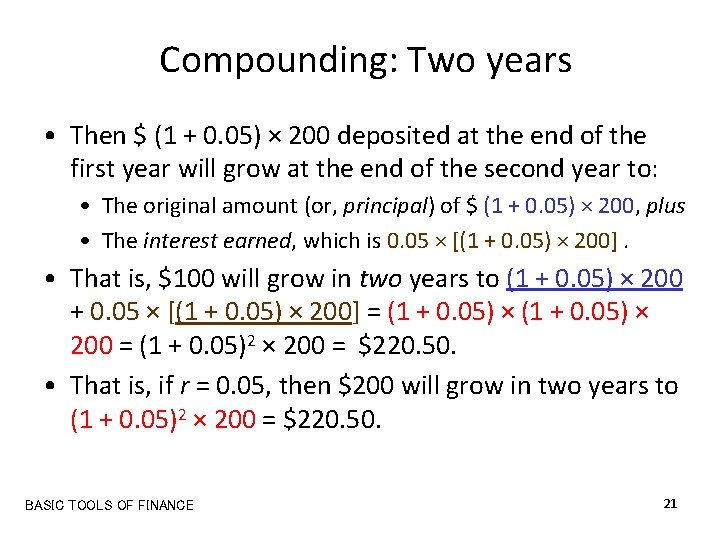Compounding: Two years • Then \$ (1 + 0. 05) × 200 deposited at the end of the first year will grow at the end of the second year to: • The original amount (or, principal) of \$ (1 + 0. 05) × 200, plus • The interest earned, which is 0. 05 × [(1 + 0. 05) × 200]. • That is, \$100 will grow in two years to (1 + 0. 05) × 200 + 0. 05 × [(1 + 0. 05) × 200] = (1 + 0. 05) × 200 = (1 + 0. 05)2 × 200 = \$220. 50. • That is, if r = 0. 05, then \$200 will grow in two years to (1 + 0. 05)2 × 200 = \$220. 50. BASIC TOOLS OF FINANCE 21 Copyright © 2004 South-Western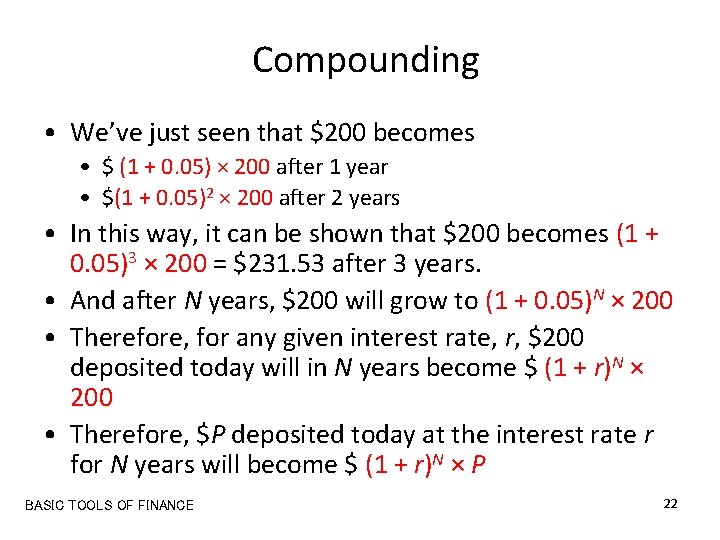Compounding • We’ve just seen that \$200 becomes • \$ (1 + 0. 05) × 200 after 1 year • \$(1 + 0. 05)2 × 200 after 2 years • In this way, it can be shown that \$200 becomes (1 + 0. 05)3 × 200 = \$231. 53 after 3 years. • And after N years, \$200 will grow to (1 + 0. 05)N × 200 • Therefore, for any given interest rate, r, \$200 deposited today will in N years become \$ (1 + r)N × 200 • Therefore, \$P deposited today at the interest rate r for N years will become \$ (1 + r)N × P BASIC TOOLS OF FINANCE 22 Copyright © 2004 South-WesternFuture Value Formula • That is, if \$P deposited today at the interest rate r becomes \$F after N years, then F = (1 + r)N × P • Recall from the definitions of future value and present value, that if \$P deposited today becomes \$F after N years, then, • \$F is the future value of \$P, and • \$P is the present value of \$F. BASIC TOOLS OF FINANCE 23 Copyright © 2004 South-WesternPresent Value Formula • But F = (1 + r)N × P implies that P = F / (1 + r)N. • Therefore, the present value of \$F to be received N years in the future is P = F / (1 + r)N BASIC TOOLS OF FINANCE 24 Copyright © 2004 South-WesternFuture value and present value • To repeat, • If we know P, the present value, we can calculate its future value as F = (1 + r)N × P. • And if we know F, the future value, we can calculate its present value as P = F / (1 + r)N. BASIC TOOLS OF FINANCE 25 Copyright © 2004 South-WesternExamples: future values and present values • If the interest rate is r = 0. 10 (or 10%), the future value of \$200 after 8 years can be calculated as F = (1 + r)N × P = (1. 10)8 × 200 = \$428. 72 • If the interest rate is r = 0. 08 (or 8%), the present value of \$500 to be received after 10 years can be calculated as P = F / (1 + r)N = 500/(1. 08)10 = \$231. 60 BASIC TOOLS OF FINANCE 26 Copyright © 2004 South-Western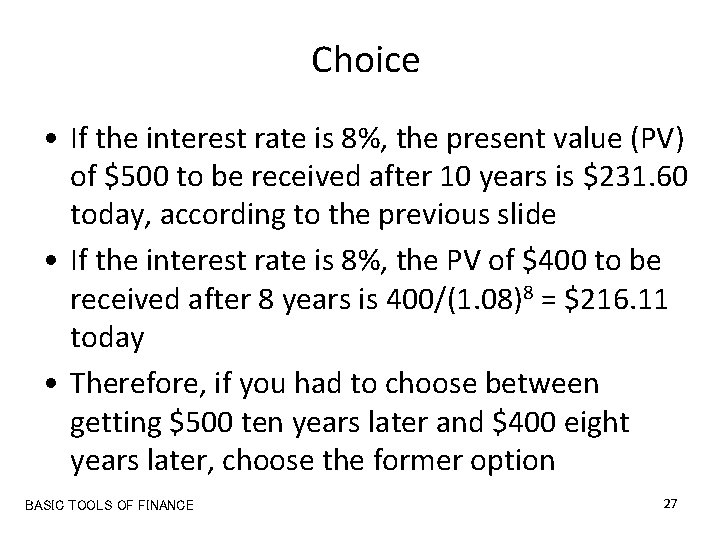Choice • If the interest rate is 8%, the present value (PV) of \$500 to be received after 10 years is \$231. 60 today, according to the previous slide • If the interest rate is 8%, the PV of \$400 to be received after 8 years is 400/(1. 08)8 = \$216. 11 today • Therefore, if you had to choose between getting \$500 ten years later and \$400 eight years later, choose the former option BASIC TOOLS OF FINANCE 27 Copyright © 2004 South-WesternChoice • If you had to choose between • Getting \$500 ten years later plus \$400 eight years later, or • Getting \$450 today, • Which option would you choose? • If r = 0. 08, the total PV of the first option is 231. 60 + 216. 11 = \$447. 71 and the PV of the second option is, obviously, \$450. • So, choose the second option because it has the higher PV. BASIC TOOLS OF FINANCE 28 Copyright © 2004 South-Western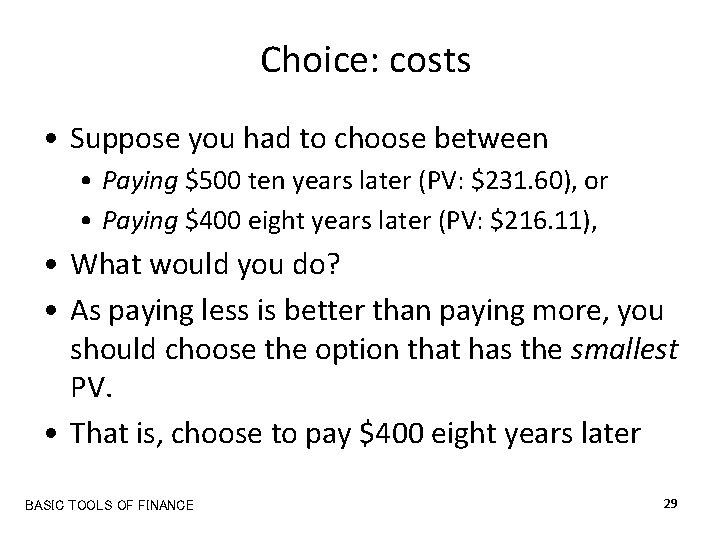Choice: costs • Suppose you had to choose between • Paying \$500 ten years later (PV: \$231. 60), or • Paying \$400 eight years later (PV: \$216. 11), • What would you do? • As paying less is better than paying more, you should choose the option that has the smallest PV. • That is, choose to pay \$400 eight years later BASIC TOOLS OF FINANCE 29 Copyright © 2004 South-WesternChoice: investments • Suppose you were offered the following investment opportunity by your friend: • You pay \$440 today (PV: \$440) to your friend • Your friend will pay you \$400 eight years later (PV: \$216. 11), plus • \$500 ten years later (PV: \$231. 60). • Should you accept his offer? • If r = 0. 08, the total PV of the receipts is 231. 60 + 216. 11 = \$447. 71, which exceeds the PV of your costs. • So, you should accept the offer. BASIC TOOLS OF FINANCE 30 Copyright © 2004 South-WesternPRESENT VALUE: MEASURING THE TIME VALUE OF MONEY • The concept of present value demonstrates the following: • Receiving a given sum of money in the present is preferred to receiving the same sum in the future. • In order to compare values at different points in time, compare their present values. • Firms undertake investment projects if the present value of the project exceeds the cost.Present value and the interest rate • Recall the present value formula: P = F / (1 + r)N. • Therefore, when either r or N increases, the present value decreases. • The bigger the delay in the receipt of an amount of money, the less attractive it is to you today • The higher the interest rate, the less attractive it is to have to wait because you know that if you had the money now you could have put it in a bank and earned the higher interest rate. BASIC TOOLS OF FINANCE 32 Copyright © 2004 South-Western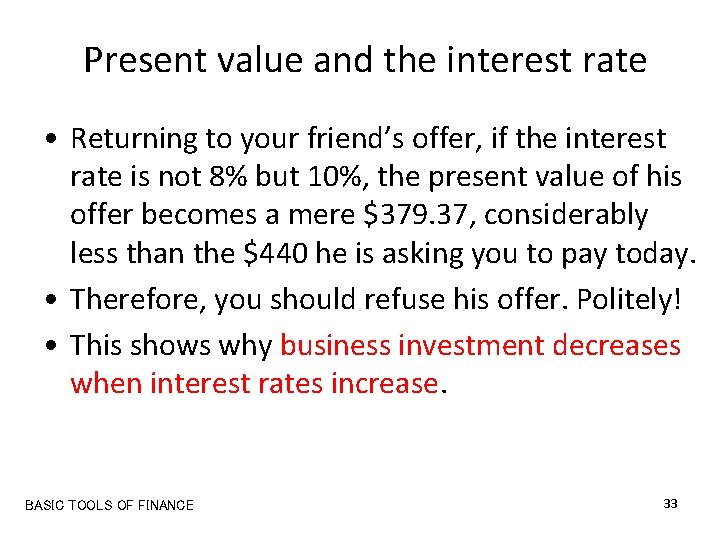Present value and the interest rate • Returning to your friend’s offer, if the interest rate is not 8% but 10%, the present value of his offer becomes a mere \$379. 37, considerably less than the \$440 he is asking you to pay today. • Therefore, you should refuse his offer. Politely! • This shows why business investment decreases when interest rates increase. BASIC TOOLS OF FINANCE 33 Copyright © 2004 South-Western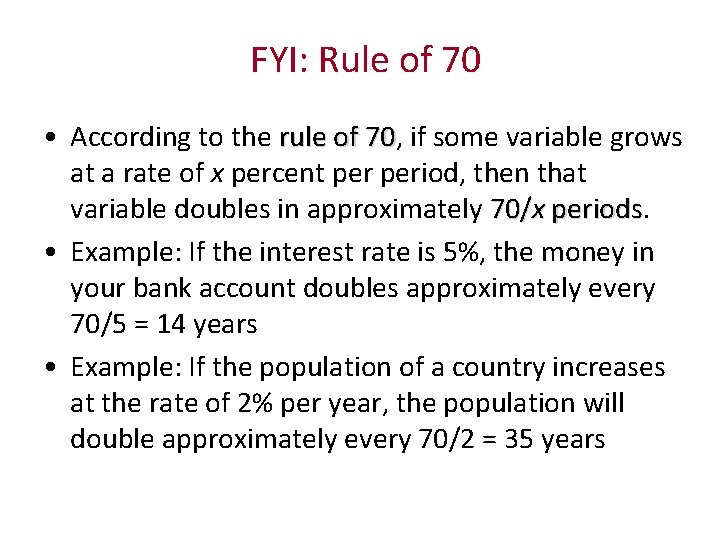FYI: Rule of 70 • According to the rule of 70, 70 if some variable grows at a rate of x percent period, then that variable doubles in approximately 70/x periods • Example: If the interest rate is 5%, the money in your bank account doubles approximately every 70/5 = 14 years • Example: If the population of a country increases at the rate of 2% per year, the population will double approximately every 70/2 = 35 years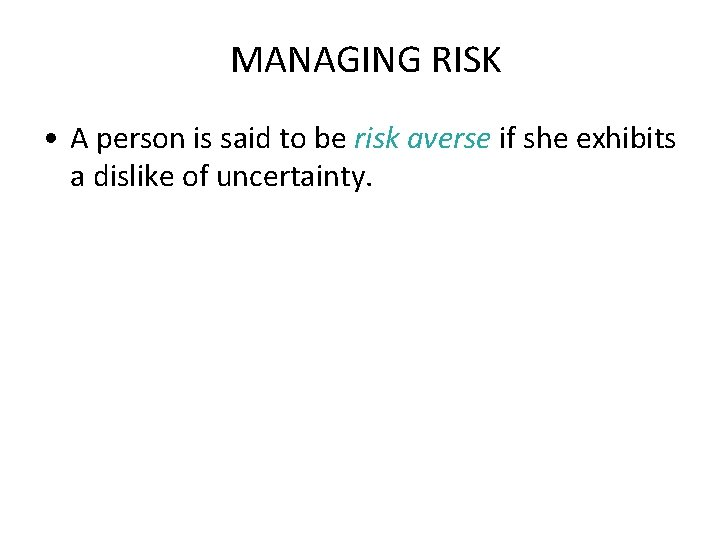MANAGING RISK • A person is said to be risk averse if she exhibits a dislike of uncertainty.MANAGING RISK • Individuals can reduce risk by choosing to do any of the following: • Buy insurance • Diversify the assets they own • Accept a lower return on their investments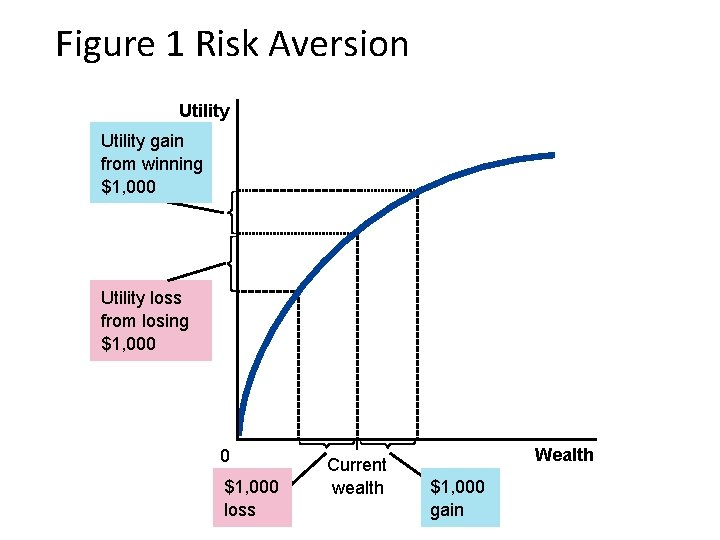Figure 1 Risk Aversion Utility gain from winning \$1, 000 Utility loss from losing \$1, 000 0 \$1, 000 loss Current wealth Wealth \$1, 000 gainThe Markets for Insurance • One way to deal with risk is to buy insurance. • The general feature of insurance contracts is that a person facing a risk pays a fee to an insurance company, which in return agrees to accept all or part of the risk.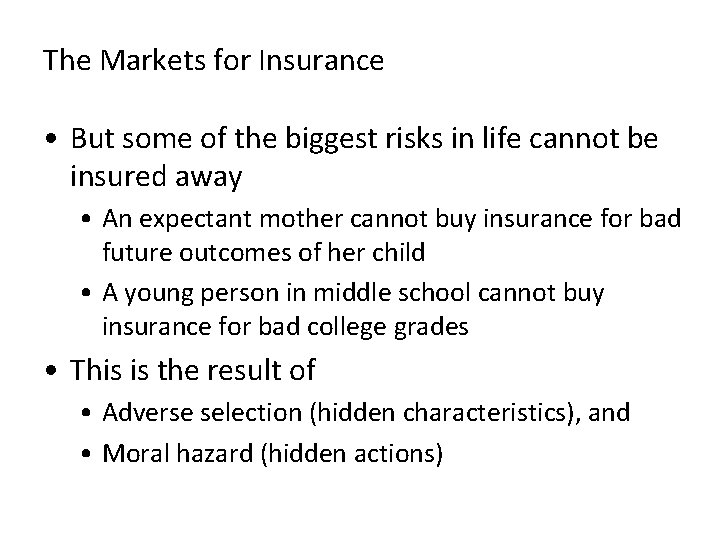The Markets for Insurance • But some of the biggest risks in life cannot be insured away • An expectant mother cannot buy insurance for bad future outcomes of her child • A young person in middle school cannot buy insurance for bad college grades • This is the result of • Adverse selection (hidden characteristics), and • Moral hazard (hidden actions)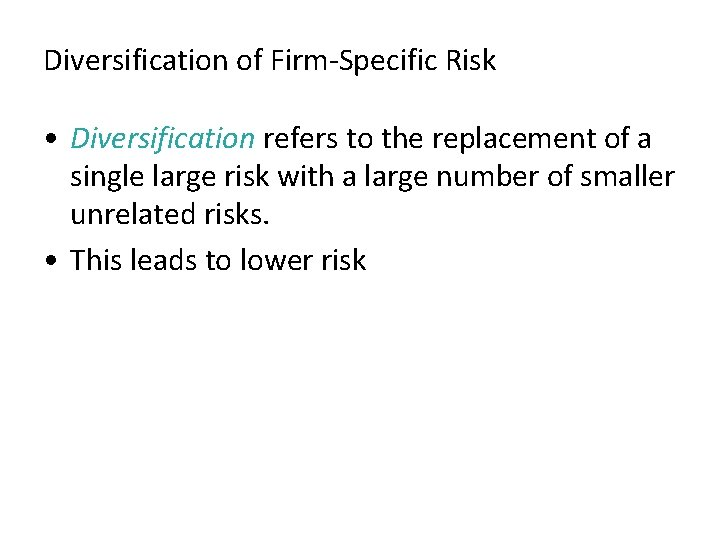Diversification of Firm-Specific Risk • Diversification refers to the replacement of a single large risk with a large number of smaller unrelated risks. • This leads to lower risk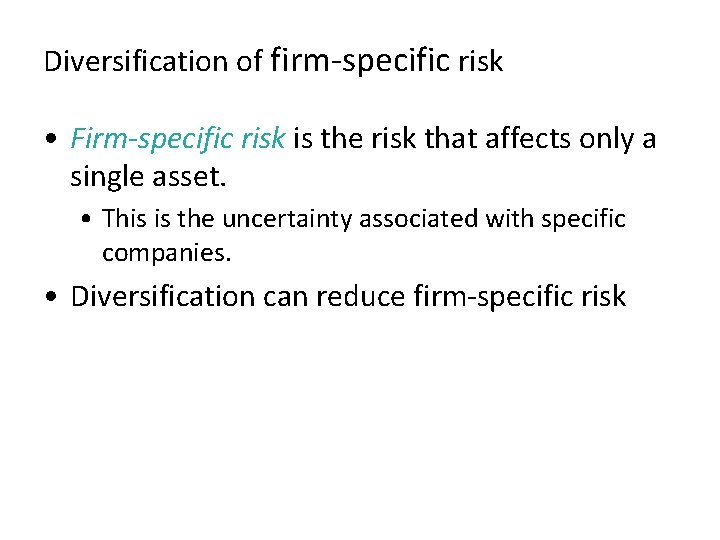Diversification of firm-specific risk • Firm-specific risk is the risk that affects only a single asset. • This is the uncertainty associated with specific companies. • Diversification can reduce firm-specific risk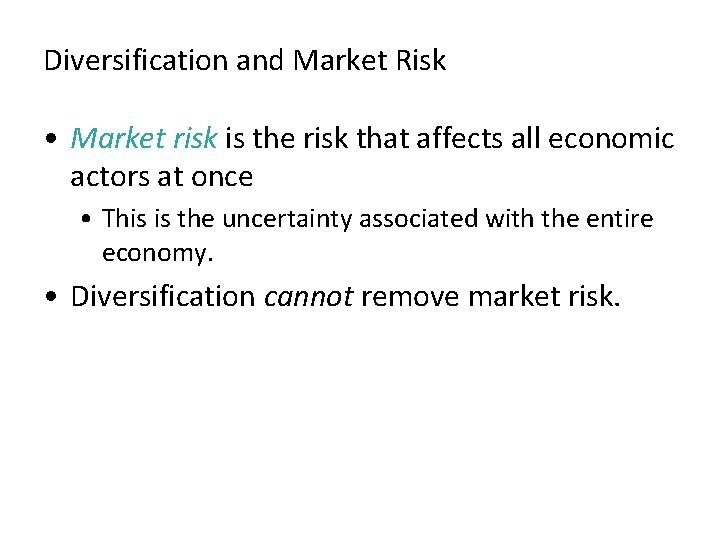Diversification and Market Risk • Market risk is the risk that affects all economic actors at once • This is the uncertainty associated with the entire economy. • Diversification cannot remove market risk.Figure 2 Diversification Risk (standard deviation of portfolio return) 1. Increasing the number of stocks in a portfolio reduces firm-specific risk through diversification… (More risk) 49 2. …but market risk remains. 20 (Less risk) 0 1 4 6 8 10 BASIC TOOLS OF FINANCE 20 30 40 Number of Stocks in Portfolio 43 Copyright © 2004 South-Western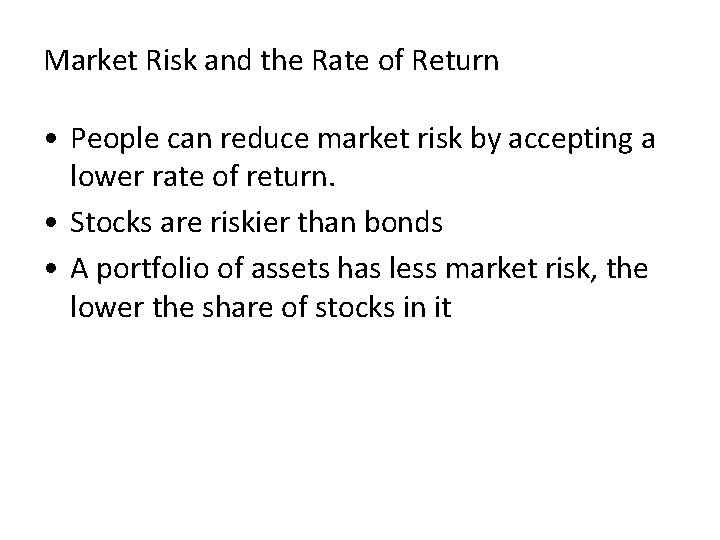Market Risk and the Rate of Return • People can reduce market risk by accepting a lower rate of return. • Stocks are riskier than bonds • A portfolio of assets has less market risk, the lower the share of stocks in it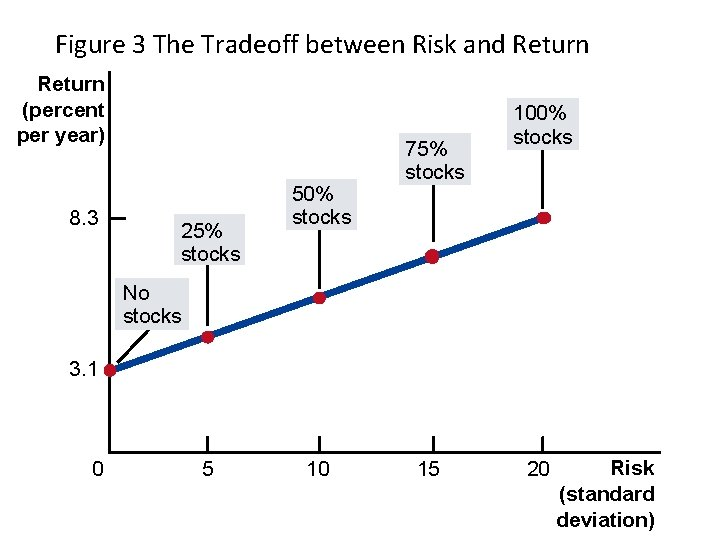Figure 3 The Tradeoff between Risk and Return (percent per year) 8. 3 25% stocks 50% stocks 75% stocks 100% stocks No stocks 3. 1 0 5 10 15 20 Risk (standard deviation)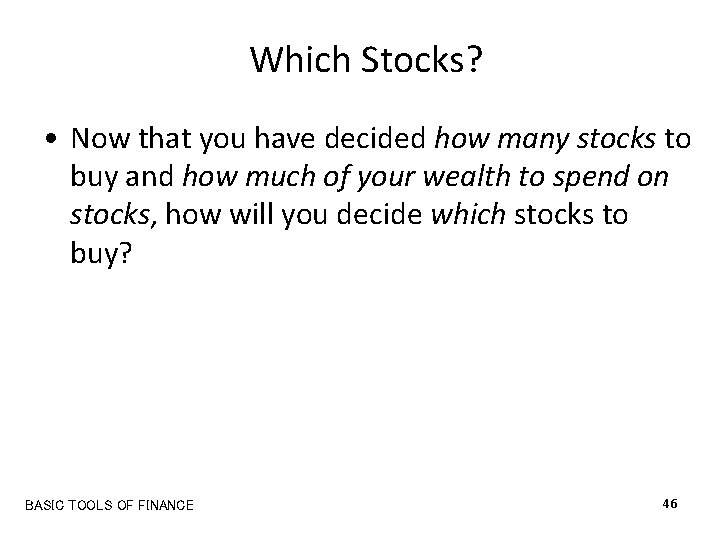Which Stocks? • Now that you have decided how many stocks to buy and how much of your wealth to spend on stocks, how will you decide which stocks to buy? BASIC TOOLS OF FINANCE 46 Copyright © 2004 South-Western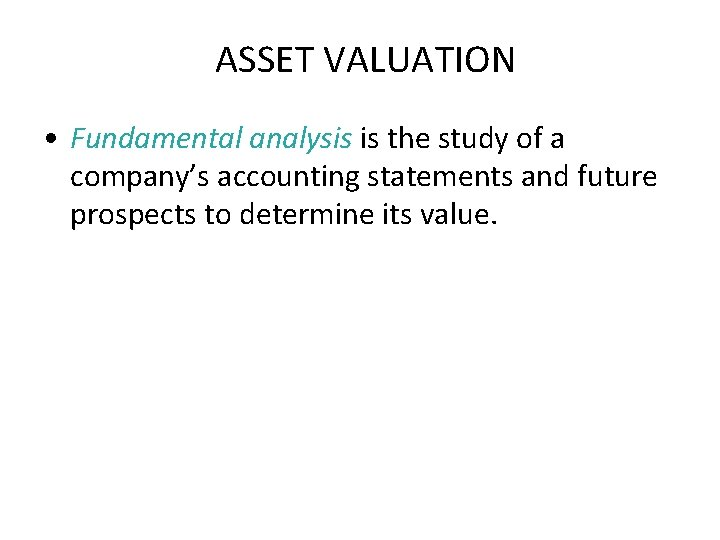ASSET VALUATION • Fundamental analysis is the study of a company’s accounting statements and future prospects to determine its value.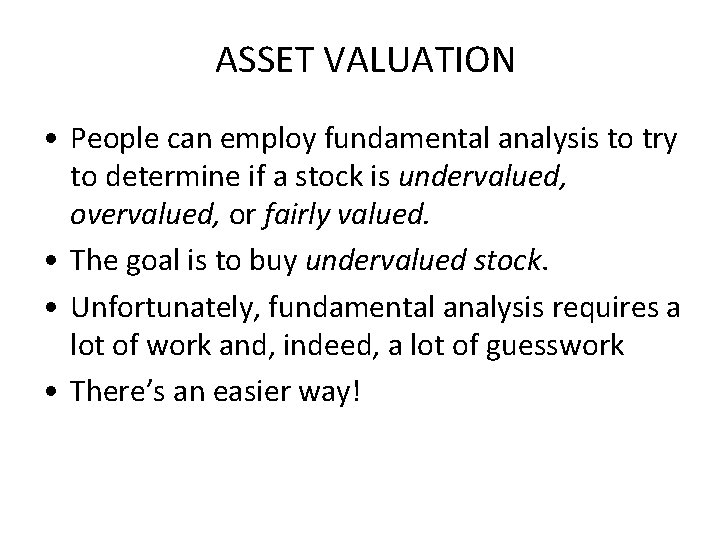ASSET VALUATION • People can employ fundamental analysis to try to determine if a stock is undervalued, overvalued, or fairly valued. • The goal is to buy undervalued stock. • Unfortunately, fundamental analysis requires a lot of work and, indeed, a lot of guesswork • There’s an easier way!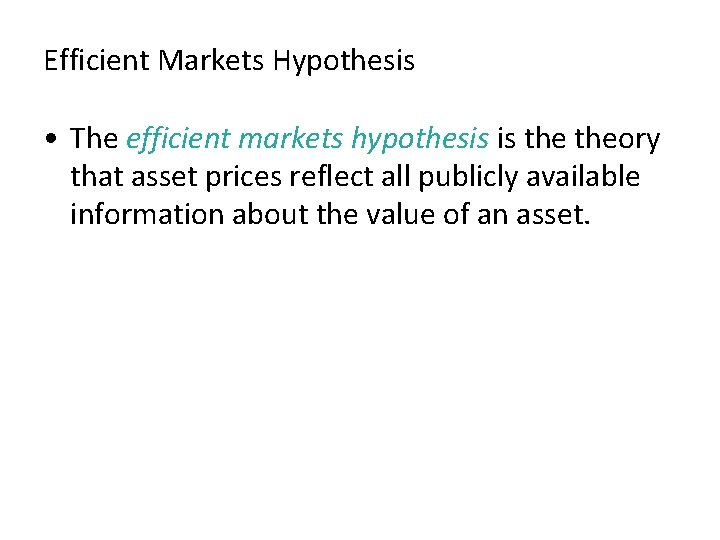Efficient Markets Hypothesis • The efficient markets hypothesis is theory that asset prices reflect all publicly available information about the value of an asset.Efficient Markets Hypothesis • A market is informationally efficient when it reflects all available information in a rational way. • If markets are efficient, the only wise thing an investor can do is buy a diversified portfolio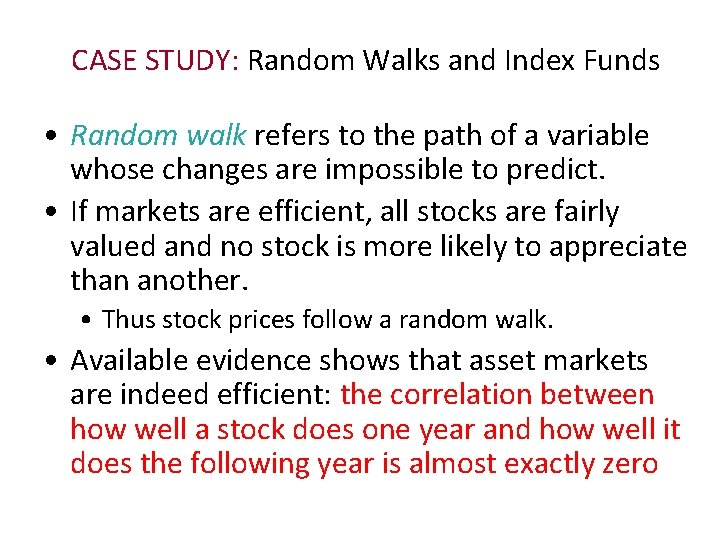CASE STUDY: Random Walks and Index Funds • Random walk refers to the path of a variable whose changes are impossible to predict. • If markets are efficient, all stocks are fairly valued and no stock is more likely to appreciate than another. • Thus stock prices follow a random walk. • Available evidence shows that asset markets are indeed efficient: the correlation between how well a stock does one year and how well it does the following year is almost exactly zero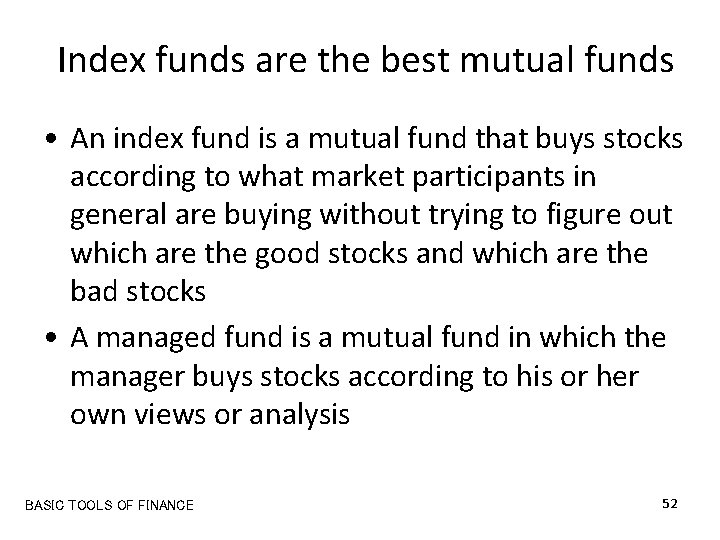Index funds are the best mutual funds • An index fund is a mutual fund that buys stocks according to what market participants in general are buying without trying to figure out which are the good stocks and which are the bad stocks • A managed fund is a mutual fund in which the manager buys stocks according to his or her own views or analysis BASIC TOOLS OF FINANCE 52 Copyright © 2004 South-Western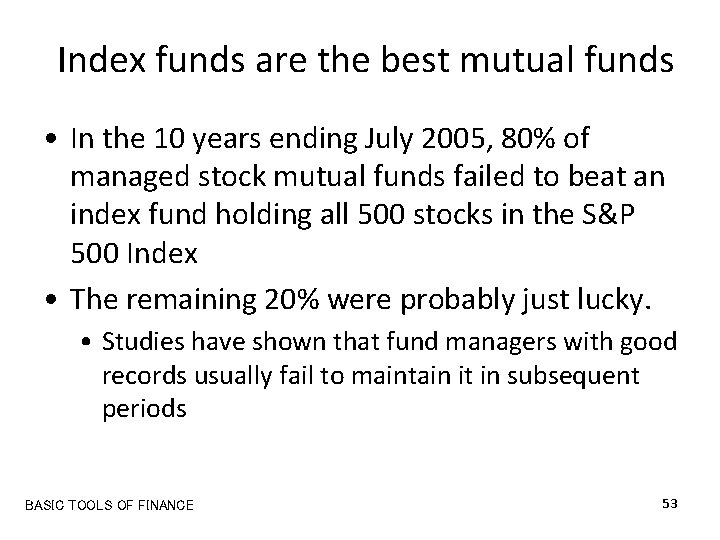Index funds are the best mutual funds • In the 10 years ending July 2005, 80% of managed stock mutual funds failed to beat an index fund holding all 500 stocks in the S&P 500 Index • The remaining 20% were probably just lucky. • Studies have shown that fund managers with good records usually fail to maintain it in subsequent periods BASIC TOOLS OF FINANCE 53 Copyright © 2004 South-WesternIndex funds are the best mutual funds • So, what’s the best strategy? • First, decide how much of your money you wish to put in stocks and in bonds • Then spend those amounts on an index fund for stocks and an index fund for bonds. • Done! • Want to be more sophisticated? Buy a domestic index fund a foreign index fund in each category • Whatever you do, don’t pay high fees for the advice of a so-called expert BASIC TOOLS OF FINANCE 54 Copyright © 2004 South-WesternSummary • Because savings can earn interest, a sum of money today is more valuable than the same sum of money in the future. • A person can compare sums from different times using the concept of present value. • The present value of any future sum is the amount that would be needed today, given prevailing interest rates, to produce the future sum.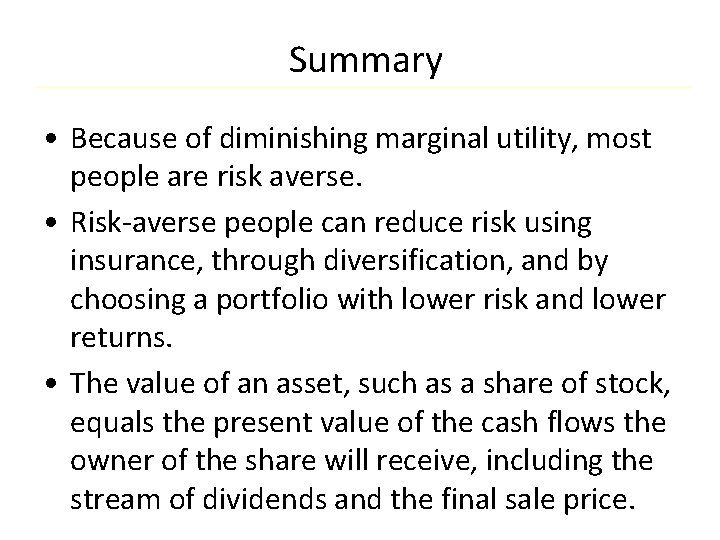Summary • Because of diminishing marginal utility, most people are risk averse. • Risk-averse people can reduce risk using insurance, through diversification, and by choosing a portfolio with lower risk and lower returns. • The value of an asset, such as a share of stock, equals the present value of the cash flows the owner of the share will receive, including the stream of dividends and the final sale price.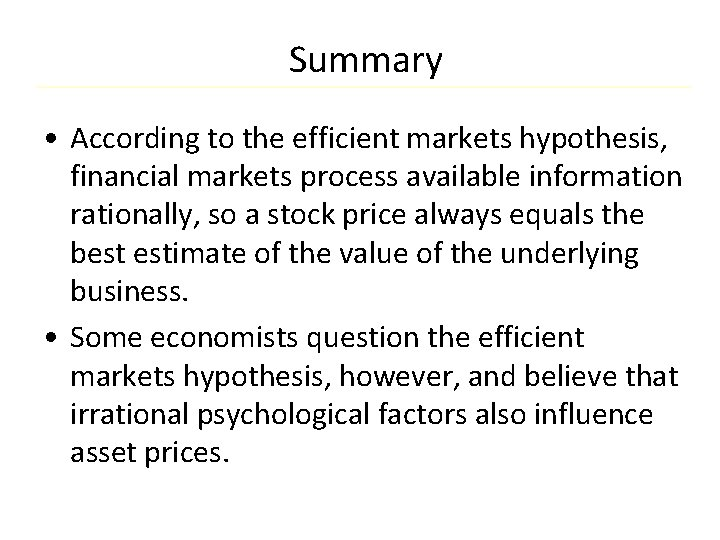Summary • According to the efficient markets hypothesis, financial markets process available information rationally, so a stock price always equals the best estimate of the value of the underlying business. • Some economists question the efficient markets hypothesis, however, and believe that irrational psychological factors also influence asset prices.